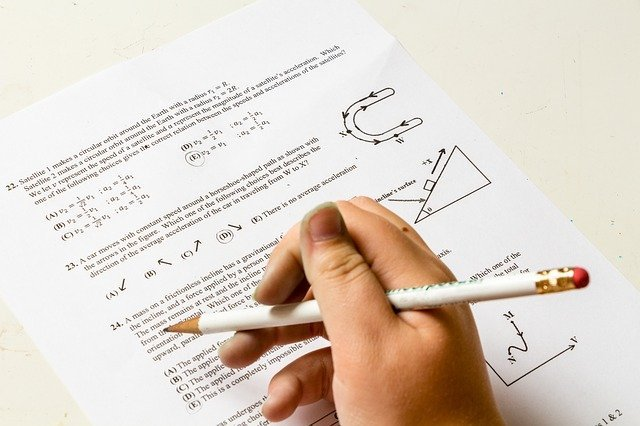# What are complex numbers?

0
284Mathematics is of keen importance since ancient times. It helps not only in the business or banking sector but also in the architecture field. Archimedes is known as the father of mathematics as he gave the concept of geometry and made varieties of machines like pulleys.

The discipline of mathematics makes our life orderly and encourages one’s problem-solving ability, critical thinking, etc. Nowadays, many competitive exams include mathematics as a crucial part of their syllabus as it provides an effective way of building mental discipline.

The subject contains algebra, geometry, complex numbers, integer, trigonometry, polynomials, matrices, etc. Understanding the topic is necessary to gain perfection and interest in the subject.

Complex numbers is one of the main chapters to understand mathematics in-depth. It is the part of various mathematical exams so, Information regarding complex numbers are listed below-

• What are complex numbers?  – complex numbers do not mean that they are complicated, it means combining two types of numbers – a real number and an imaginary number. The standardized form of complex numbers is a+ib, where a = real number and ib = imaginary number, Here i = √-1. They can be applied in real life in various phases, like electronics and electromagnetism.
• What are real numbers? – Real numbers comprise positive numbers, negative numbers, zero, integer, fractions, etc. they are well-presented in the number system as Re(). For example- 7, -90, 1/4, etc.
• What are imaginary numbers? – It is defined as the square root of a negative number and presented as Im().  It always gives negative results when one multiplies them. It also contains an imaginary unit – “i”. For example- 5i, 7i, etc.
• Graphical representation – Graph has two-axis: (x-axis and y-axis). The X-axis represents the real number and the y-axis represents an imaginary number.
• Modulus and conjugate – let X = p+iq be a complex number.Here modulus of X represented by |X| = √p^2+q^2, mathematically.

Conjugate of X = p-iq.

• Rules regarding complex numbers – Addition, Subtraction, Multiplication, Division are the four types of algebraic operations on complex numbers. The real number must perform the algebraic operation with the real number and the same for an imaginary number.
1. Addition: (p + iq) + (r + is) = (p + r) +i(q + s)
2. subtraction: (p + iq) – (r + is) = (p – r ) +i(q – s)
3. Multiplication: (p + iq). (r + is) = (pr – qs) +i(ps + qr)
4. Division: Multiplying the numerator and denominator by the denominator’s conjugate value. (p + iq) / (r + is) = (pr + qs) / (r^2 + s^2) + i(qr – ps) / (r^2 + s^2).
• Properties of complex numbers –
1. The multiplication and addition of two conjugate numbers will give a real number.